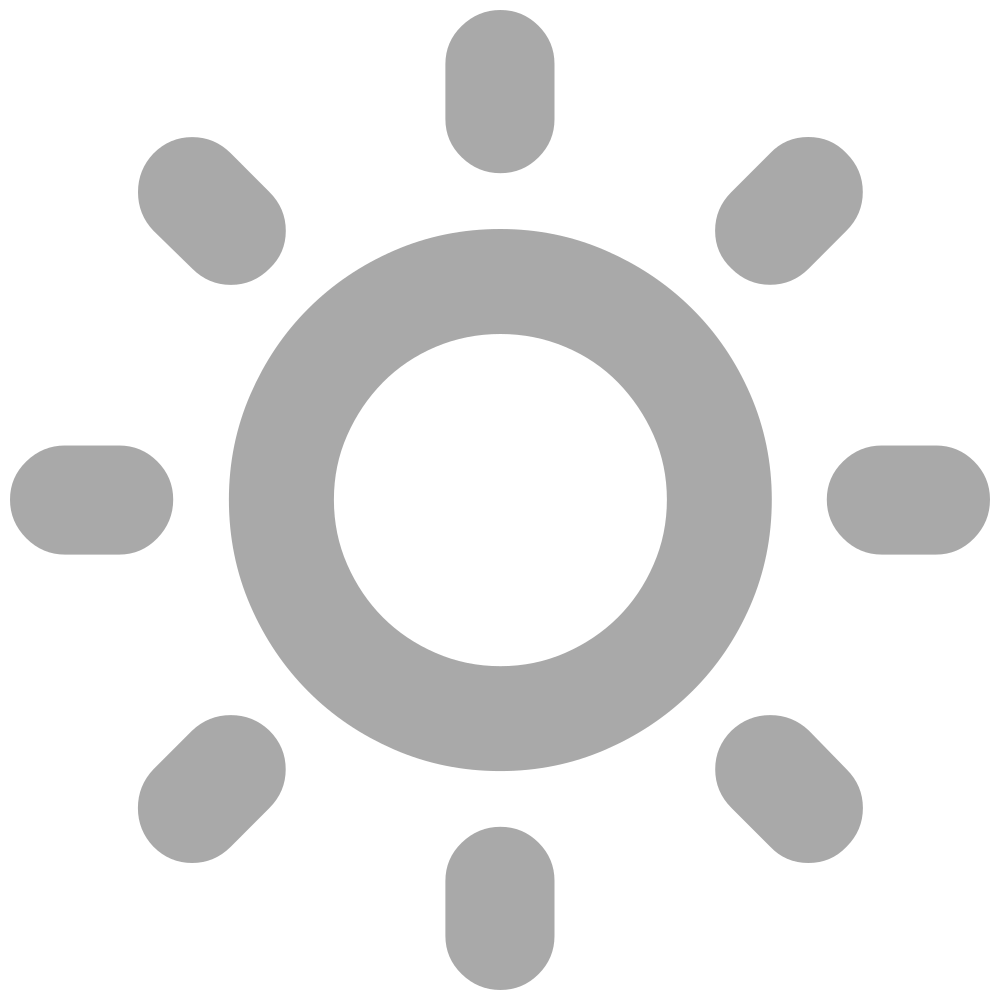Nuggets# Tagged with Modeling

## V. I. Arnold on mathematics as an experimental science

The mathematician V. I. Arnold delivered an interesting (and quite opinionated) speech in 1997 (archive).

Here are some passages about math and physics that caught my attention.

On mathematics being an experimental science:

Mathematics is a part of physics. Physics is an experimental science, a part of natural science. Mathematics is the part of physics where experiments are cheap.

The Jacobi identity (which forces the heights of a triangle to cross at one point) is an experimental fact in the same way as that the Earth is round (that is, homeomorphic to a ball). But it can be discovered with less expense.

According to his viewpoint, math develops first with observations, then with the effort to find the limits of the observations, then with attempt to generalize what holds up through a conjecture, then with the “modeling” using formal logic:

The scheme of construction of a mathematical theory is exactly the same as that in any other natural science. First we consider some objects and make some observations in special cases. Then we try and find the limits of application of our observations, look for counter-examples which would prevent unjustified extension of our observations onto a too wide range of events (example: the number of partitions of consecutive odd numbers $1, 3, 5, 7, 9$ into an odd number of natural summands gives the sequence $1, 2, 4, 8, 16$, but then comes $29$).

As a result we formulate the empirical discovery that we made (for example, the Fermat conjecture or Poincaré conjecture) as clearly as possible. After this there comes the difficult period of checking as to how reliable are the conclusions.

At this point a special technique has been developed in mathematics. This technique, when applied to the real world, is sometimes useful, but can sometimes also lead to self-deception. This technique is called modelling. When constructing a model, the following idealisation is made: certain facts which are only known with a certain degree of probability or with a certain degree of accuracy, are considered to be “absolutely” correct and are accepted as “axioms”. The sense of this “absoluteness” lies precisely in the fact that we allow ourselves to use these “facts” according to the rules of formal logic, in the process declaring as “theorems” all that we can derive from them.

On the perils of getting too far away from the “reality”:

It is obvious that in any real-life activity it is impossible to wholly rely on such deductions. The reason is at least that the parameters of the studied phenomena are never known absolutely exactly and a small change in parameters (for example, the initial conditions of a process) can totally change the result. Say, for this reason a reliable long-term weather forecast is impossible and will remain impossible, no matter how much we develop computers and devices which record initial conditions.

In exactly the same way a small change in axioms (of which we cannot be completely sure) is capable, generally speaking, of leading to completely different conclusions than those that are obtained from theorems which have been deduced from the accepted axioms. The longer and fancier is the chain of deductions (“proofs”), the less reliable is the final result.

Complex models are rarely useful (unless for those writing their dissertations).

The mathematical technique of modelling consists of ignoring this trouble and speaking about your deductive model in such a way as if it coincided with reality. The fact that this path, which is obviously incorrect from the point of view of natural science, often leads to useful results in physics is called “the inconceivable effectiveness of mathematics in natural sciences” — or “the Wigner principle”.

Here we can add a remark by I. M. Gel’fand: there exists yet another phenomenon which is comparable in its inconceivability with the inconceivable effectiveness of mathematics in physics noted by Wigner — this is the equally inconceivable ineffectiveness of mathematics in biology.

The subtle poison of mathematical education (in Felix Klein’s words) for a physicist consists precisely in that the absolutised model separates from the reality and is no longer compared with it. Here is a simple example: mathematics teaches us that the solution of the Malthus equation, $dx/dt=x$, is uniquely defined by the initial conditions (that is that the corresponding integral curves in the $(t,x)$-plane do not intersect each other). This conclusion of the mathematical model bears little relevance to the reality. A computer experiment shows that all these integral curves have common points on the negative $t$-semi-axis. Indeed, say, curves with the initial conditions $x(0)=0$ and $x(0)=1$ practically intersect at $t=−10$ and at $t=−100$. You cannot fit in an atom between them. Properties of the space at such small distances are not described at all by Euclidean geometry. Application of the uniqueness theorem in this situation obviously exceeds the accuracy of the model. This has to be respected in practical application of the model, otherwise one might find oneself faced with serious troubles.

I would like to note, however, that the same uniqueness theorem explains why the closing stage of mooring of a ship to the quay is carried out manually: on steering, if the velocity of approach would have been defined as a smooth (linear) function of the distance, the process of mooring would have required an infinitely long period of time. An alternative is an impact with the quay (which is damped by suitable non-ideally elastic bodies). By the way, this problem had to be seriously confronted on landing the first descending apparata on the Moon and Mars and also on docking with space stations — here the uniqueness theorem is working against us.